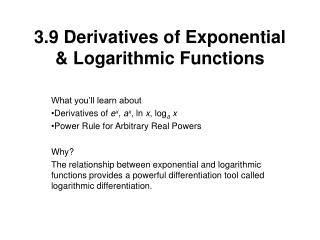DownloadDownload Presentation3.9 Derivatives of Exponential & Logarithmic Functions

# 3.9 Derivatives of Exponential & Logarithmic Functions

Télécharger la présentation## 3.9 Derivatives of Exponential & Logarithmic Functions

- - - - - - - - - - - - - - - - - - - - - - - - - - - E N D - - - - - - - - - - - - - - - - - - - - - - - - - - -
##### Presentation Transcript

1. 3.9 Derivatives of Exponential & Logarithmic Functions What you’ll learn about Derivatives of ex, ax, ln x, logax Power Rule for Arbitrary Real Powers Why? The relationship between exponential and logarithmic functions provides a powerful differentiation tool called logarithmic differentiation.

2. Derivatives Copy onto derivative sheet, then memorize.

3. Let’s use these formulas! Using find Using find

4. At what point on the graph of the function y=3t – 4 does the tangent line have slope 12? How can we find this out? • Find dy/dt and set it equal to zero. • Solve for t. • Evaluate f(t) in the original equation. • Hint: t is a messy number, store value for accuracy in final answer.

5. A line with slope m=1/a passes through the origin and is tangent to the graph of y = ln x. What is the value of m? We know • Point of tangency has coordinates (a, ln a) for some positive a • Tangent line has slope m = 1/a • Tangent line passes through the origin so equation has point (0, 0) Find slope with formula and set it equal to 1/a. Solve for a.

6. Homework Page 178 Quick Review 1-10 Exercise 3-21 (3n, nЄI)

7. Going the long way with the chain rule! Find a = u(x)= u’(x) =

8. You try a = u = u’ =

9. Using the power rule in all of its Power! Find each derivative using

10. What does it mean to “Find Domain”? If f(x) = ln (x - 6), find f ’(x). State the domain of f ’. • Find f ’ • Where is f ’ defined?

11. Sometimes we should use logarithms to simplify before differentiation. Find dy/dx for y = x x • Use logs to lose the exponent, THEN take the derivative implicitly.

12. How Fast does H1N1 Spread? The spread of a flu in a certain school is modeled by the equation Where P(t) is the total number of students infected t days after the flu was first noticed. Many of them may already be well again at time t. • Estimate the initial number of students infected with the flu. • How fast is the flu spreading after 3 days? • When will the flu spread at its maximum rate? What is this rate?

13. Homework Page 178 Exercises 24-39 (3n, nЄI), 53, 56-62 & Study for Quiz: 3.7 thru 3.9